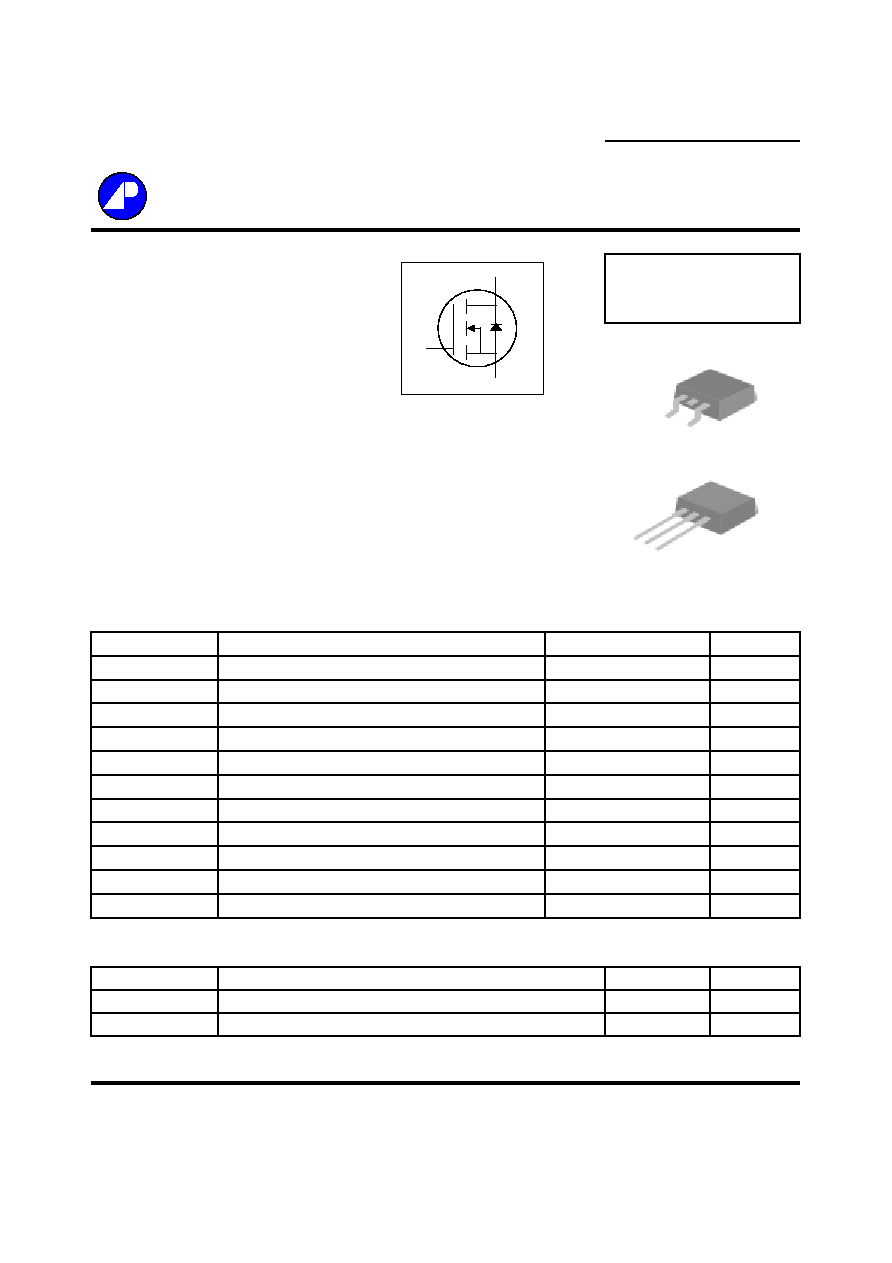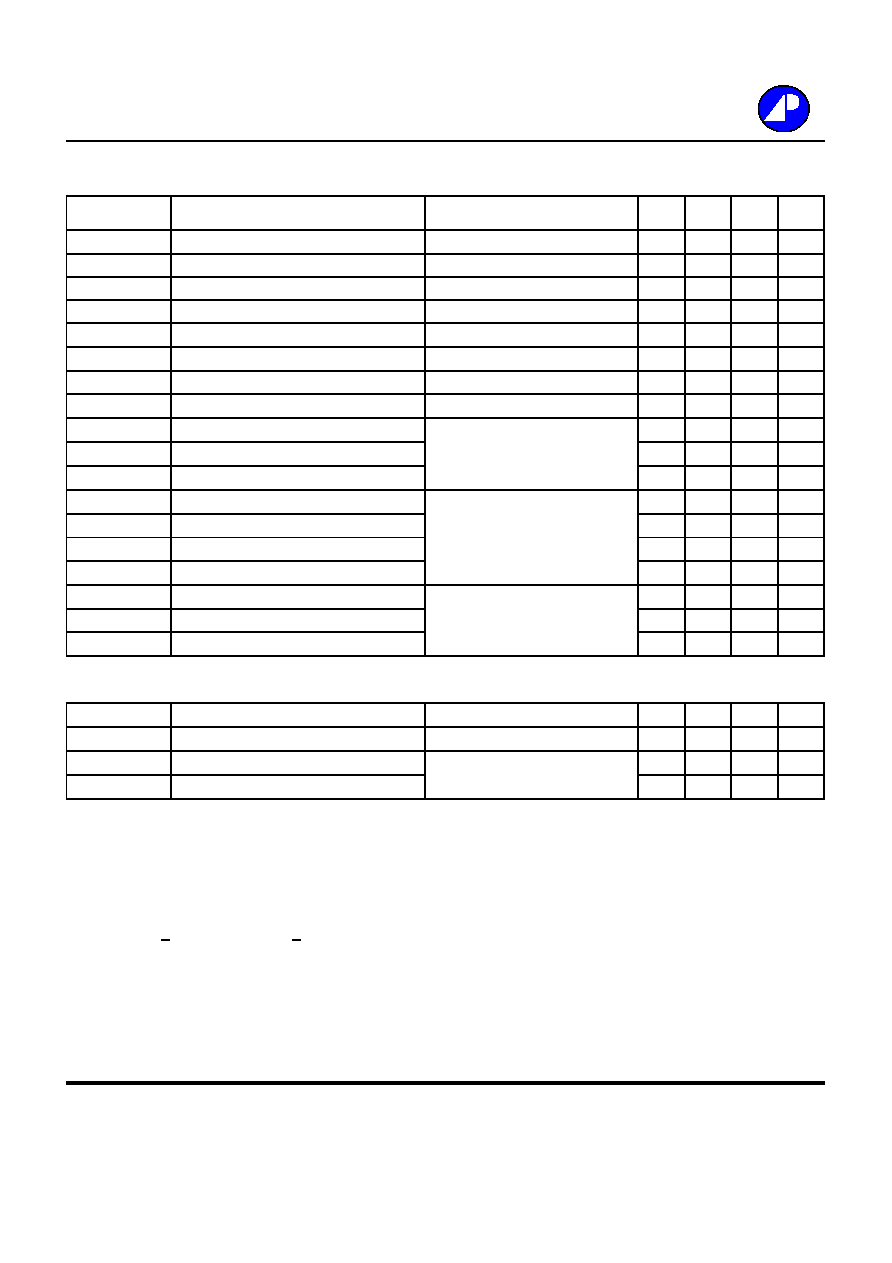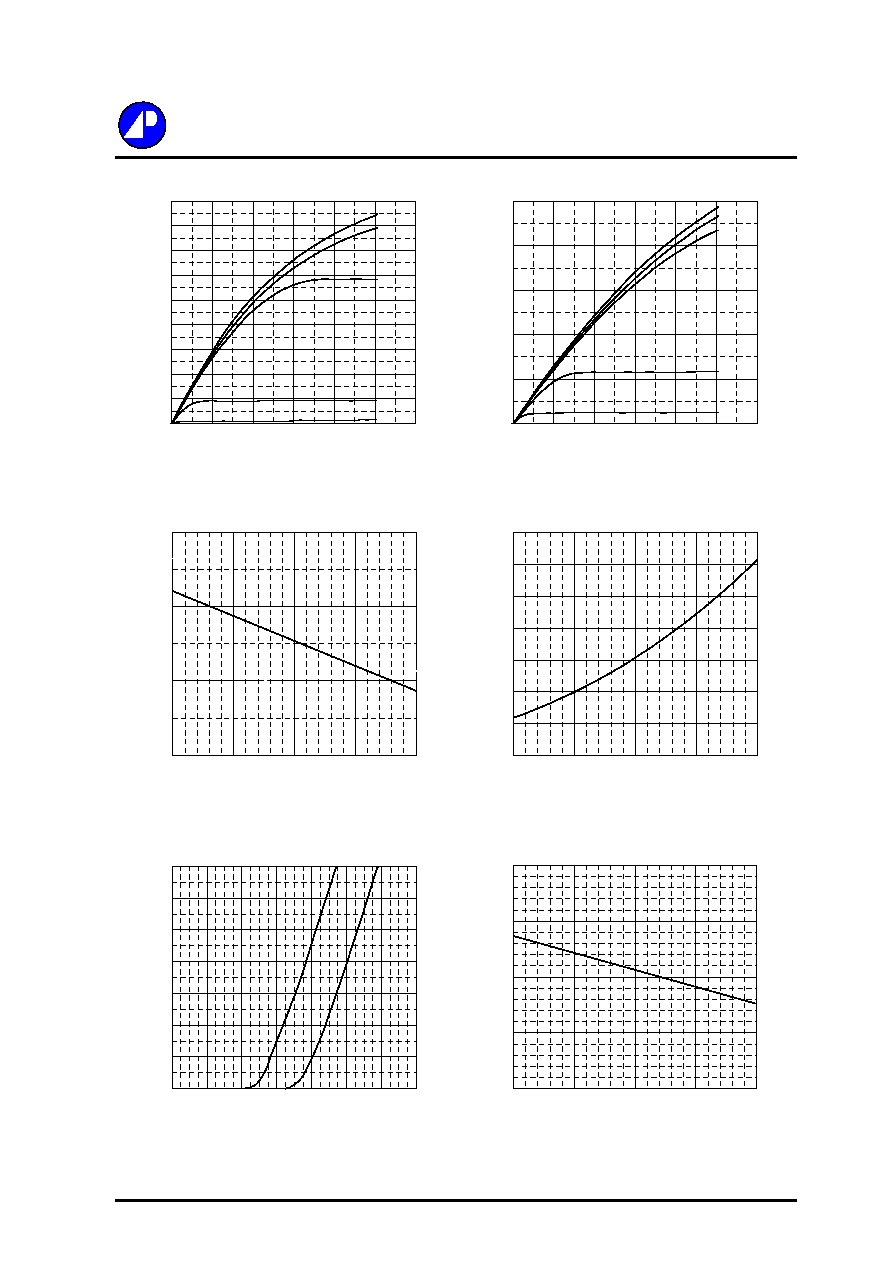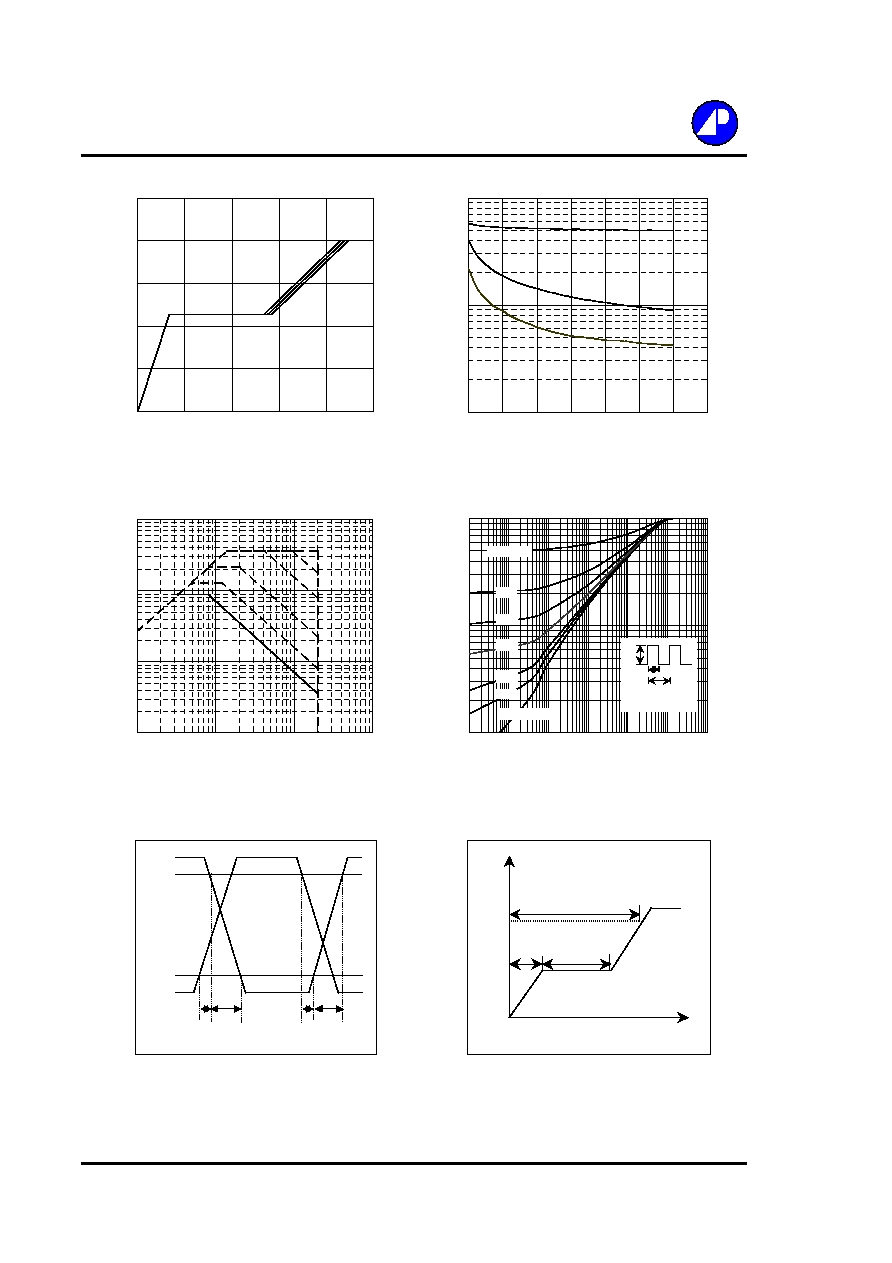Search datasheet (1.687.043 components) Search fieldPart namePart descriptionAbout site Manufacturers list ChipFindIC search engine AllXrefCross-reference database### Datasheet: AP09N20H (Advanced Power Electronics Corp.)

N-channel Enhancement Mode
Download:PDFZIPN-CHANNEL ENHANCEMENT MODE
Electronics Corp.
POWER MOSFET
Simple Drive Requirement
BV
DSS
200V
Low On-resistance
R
DS(ON)
380m
Fast Switching Characteristics
I
D
8.6A
Description
Absolute Maximum Ratings
Symbol
Units
V
DS
Drain-Source Voltage
V
V
GS
Gate-Source Voltage
V
I
D
@T
C
=25
Continuous Drain Current, V
GS
@ 10V
A
I
D
@T
C
=100
Continuous Drain Current, V
GS
@ 10V
A
I
DM
Pulsed Drain Current
1
A
P
D
@T
C
=25
Total Power Dissipation
W
W/
E
AS
Single Pulse Avalanche Energy
2
mJ
I
AR
Avalanche Current
A
T
STG
T
J
Operating Junction Temperature Range
Thermal Data
Symbol
Value
Unit
Rthj-c
Thermal Resistance Junction-case
Max.
1.8
/W
Rthj-a
Thermal Resistance Junction-ambient
Max.
110
/W
Data & specifications subject to change without notice
201112031
Storage Temperature Range
-55 to 150
36
69
Linear Derating Factor
0.55
8.6
5.5
Parameter
Rating
200
AP09N20H/J
40
-55 to 150
Parameter
8.6
30
G
D S
TO-251(J)
G D
S
TO-252(H)
The Advanced Power MOSFETs from APEC provide the
designer with the best combination of fast switching,
ruggedized device design, low on-resistance and cost-effectiveness.
The TO-252 package is universally preferred for all commercial-
industrial applications at power dissipation levels to approximately 50
watts. The through-hole version (AP09N20J) is available for low-
profile applications.
G
D
SElectrical Characteristics@T
j
=25
o
C(unless otherwise specified)
Symbol
Parameter
Test Conditions
Min.
Typ. Max. Units
BV
DSS
Drain-Source Breakdown Voltage
V
GS
=0V, I
D
=1mA
200
-
-
V
B
V
DSS
/T
j
Breakdown Voltage Temperature Coefficient
Reference to 25
, I
D
=1mA
-
0.24
-
V/
R
DS(ON)
Static Drain-Source On-Resistance
V
GS
=10V, I
D
=5A
-
-
380
m
V
GS(th)
Gate Threshold Voltage
V
DS
=V
GS
, I
D
=250uA
2
-
4
V
g
fs
Forward Transconductance
V
DS
=10V, I
D
=5A
-
3.7
-
S
I
DSS
Drain-Source Leakage Current (T
j
=25
o
C)
V
DS
=200V, V
GS
=0V
-
-
10
uA
Drain-Source Leakage Current (T
j
=150
o
C)
V
DS
=160V
,
V
GS
=0V
-
-
100
uA
I
GSS
Gate-Source Leakage
V
GS
=
-
-
nA
Q
g
Total Gate Charge
3
I
D
=8.6A
-
23
37
nC
Q
gs
Gate-Source Charge
V
DS
=160V
-
4
-
nC
Q
gd
Gate-Drain ("Miller") Charge
V
GS
=10V
-
13
-
nC
t
d(on)
Turn-on Delay Time
3
V
DD
=100V
-
12
-
ns
t
r
Rise Time
I
D
=8.6A
-
74
-
ns
t
d(off)
Turn-off Delay Time
R
G
=10
,
V
GS
=10V
-
36
-
ns
t
f
Fall Time
R
D
=11.6
-
44
-
ns
C
iss
Input Capacitance
V
GS
=0V
-
500
800
pF
C
oss
Output Capacitance
V
DS
=25V
-
90
-
pF
C
Reverse Transfer Capacitance
f=1.0MHz
-
40
-
pF
Source-Drain Diode
Symbol
Parameter
Test Conditions
Min.
Typ. Max. Units
V
SD
Forward On Voltage
3
I
S
=8.6A, V
GS
=0V
-
-
1.3
V
t
rr
Reverse Recovery Time
I
S
=8.6A, V
GS
=0V,
-
225
-
ns
Q
rr
Reverse Recovery Charge
dI/dt=100A/�s
-
2260
-
nC
Notes:
1.Pulse width limited by safe operating area.
2.Starting T
j
=25
o
C , V
DD
=50V , L=1mH , R
G
=25
, I
AS
=8.6A.
3.Pulse width <300us , duty cycle <2%.
AP09N20H/J
30V
100AP09N20H/J
Fig 1. Typical Output Characteristics
Fig 2. Typical Output Characteristics
Fig 3. Normalized BV
DSS
v.s. Junction
Fig 4. Normalized On-Resistance
Temperature
v.s. Junction Temperature
Fig 5. Forward Characteristic of
Fig 6. Gate Threshold Voltage v.s.
Reverse Diode
Junction Temperature
0
1
2
3
-50
0
50
100
150
T
j
, Junction Temperature (
o
C)
Norm
alize
d
B
V
DSS
(V)
0
0.4
0.8
1.2
1.6
2
2.4
2.8
-50
0
50
100
150
T
j
, Junction Temperature (
o
C )
Norm
alize
d
R
DS(
ON)
I
D
=5A
V
GS
=10V
0
2
4
6
8
10
12
14
16
18
0
2
4
6
8
10
12
V
DS
, Drain-to-Source Voltage (V)
I
D
, Drain Current (A)
T
C
=25
o
C
10V
8.0V
7.0V
5.0V
V
G
=4.0V
0
2
4
6
8
10
0
2
4
6
8
10
12
V
DS
, Drain-to-Source Voltage (V)
I
D
, Drain Current (A)
T
C
=150
o
C
10V
8.0V
7.0V
V
G
=4.0V
5.0V
0
1
2
3
4
5
6
7
0
0.2
0.4
0.6
0.8
1
1.2
1.4
V
SD
, Source-to-Drain Voltage (V)
I
S
(A)
T
j
=25
o
C
T
j
=150
o
C
1
2
3
4
5
-50
0
50
100
150
T
j
, Junction Temperature (
o
C )
V
GS(
t
h)
(V)AP09N20H/J
Fig 7. Gate Charge Characteristics
Fig 8. Typical Capacitance Characteristics
Fig 9. Maximum Safe Operating Area
Fig10. Effective Transient Thermal Impedance
Fig 11. Switching Time Waveform
Fig 12. Gate Charge Waveform
t
d(on)
t
r
t
d(off)
t
f
V
DS
V
GS
10%
90%
Q
V
G
10V
Q
GS
Q
GD
Q
G
Charge
10
100
1000
1
5
9
13
17
21
25
29
V
DS
, Drain-to-Source Voltage (V)
C (
p
F)
f=1.0MHz
Ciss
Coss
0
3
6
9
12
15
0
6
12
18
24
30
Q
G
, Total Gate Charge (nC)
V
GS
, Ga
te to
So
urce Vo
lta
g
e
(
V
)
V
DS
=100V
V
DS
=120V
V
DS
=160V
I
D
=8.6A
0.01
0.1
1
0.00001
0.0001
0.001
0.01
0.1
1
10
t , Pulse Width (s)
No
rm
a
lized
T
h
erm
a
l Resp
o
n
se (
R
th
jc
)
P
DM
Duty factor = t/T
Peak T
j
= P
DM
x R
thjc
+ T
C
t
T
0.02
0.01
0.05
0.1
0.2
Duty factor=0.5
Single Pulse
0
1
10
100
1
10
100
1000
V
DS
, Drain-to-Source Voltage (V)
I
D
(A
)
T
c
=25
o
C
Single Pulse
1ms
10ms
100ms
1s
DC© 2020 • ICSheet• Contact form• Main page